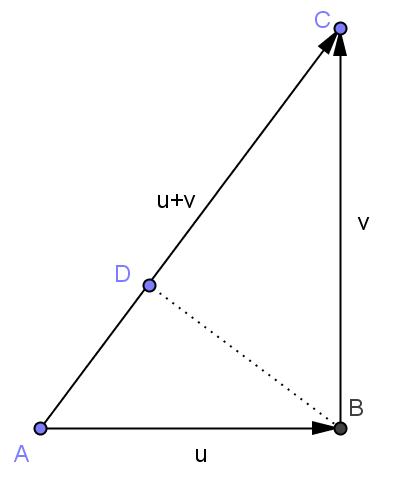# 从不同的角度看矩阵的行秩与列秩——兼论如何学好线性代数

A和A的共轭转置的列向量的秩分别对应于 T 和 T* 的值域的维度，能不能就此证明它们相等？从而至少可以证明实数矩阵行秩等于列秩。这就是下面的：

$$T^*v_1,T^*v_2,\dots,T^*v_s$$，考虑 $$TT^*v_1,TT^*v_2,\dots,TT^*v_s$$，这个向量组的秩≤s<r，因此可以在 $$T$$ 的值域中(维数为r)找到 $$v_{s+1}=Tu_{s+1}\not=0$$ 使得 $$\langle v_{s+1},TT^*v_i\rangle=\langle T^*v_{s+1},T^*v_i\rangle=0, i=1,2,\dots,s$$。又因为 $$\langle u_{s+1},T^*Tu_{s+1}\rangle=\langle Tu_{s+1},Tu_{s+1}\rangle\not=0$$ 故 $$T^*Tu_{s+1}\not=0$$ 即 $$T^*v_{s+1}\not=0$$。这样我们在 $$T^*$$ 的值域中找到了与向量 $$T^*v_1,T^*v_2,\dots,T^*v_s$$ 都垂直的非零向量，与这个向量组是 $$T^*$$ 值域的基底矛盾。因此s≥r。

$$\mathbf{a}_j=d_{1j}\mathbf{b}_1+d_{2j}\mathbf{b}_2+\cdots+d_{cj}\mathbf{b}_c$$

$$=\begin{bmatrix}\mathbf{b}_1&\mathbf{b}_2&\cdots&\mathbf{b}_c\end{bmatrix}\begin{bmatrix}d_{1j}\\d_{2j}\\\vdots\\d_{cj}\end{bmatrix}=B\mathbf{d}_j$$

$$A=\begin{bmatrix}\mathbf{a}_1&\mathbf{a}_2&\cdots&\mathbf{a}_n\end{bmatrix}=B\begin{bmatrix}\mathbf{d}_1&\mathbf{d}_2&\cdots&\mathbf{d}_n\end{bmatrix}=BD$$

$$\mathrm{row}_{i}(A)=\mathrm{row}_{i}(BD)=b_{i1}\mathrm{row}_1(D)+b_{i2}\mathrm{row}_2(D)+\cdots+b_{ic}\mathrm{row}_{c}(D)$$

$$A_{m\times n}=B_{m\times r}D_{r\times n}$$

$$\begin{bmatrix}x_1\\x_2\\\vdots\\x_r\\f_1(x_1,x_2,\dots,x_r)\\\vdots\\f_{m-r}(x_1,x_2,\dots,x_r)\end{bmatrix}$$

《理解矩阵（一）》
《理解矩阵（二）》
《理解矩阵（三）》

# 子空间和的维数定理与容斥原理

dim(U1+U2)=dimU1+dimU2-dim(U1 ∩ U2)

card(U1∪U2)=cardU1+cardU2-card(U1 ∩ U2)

card(U1∪U2∪U3)=cardU1+cardU2+cardU3-card(U1 ∩ U2)-card(U2 ∩ U3)-card(U1 ∩ U3)+card(U1 ∩ U2∩ U3)

dim(U1+U2+U3)=dimU1+dimU2+dimU3-dim(U1 ∩ U2)-dim(U2 ∩ U3)-dim(U1 ∩ U3)+dim(U1 ∩ U2∩ U3)

dim(U1+U2+U3)=dim(U1+U2)+dimU3-dim((U1+U2)∩ U3)=dimU1+dimU2+dimU3-dim(U1 ∩ U2)-dim((U1+U2)∩ U3)

dim((U1+U2)∩ U3)=dim(U1 ∩ U3)+dim(U2 ∩ U3)-dim(U1 ∩ U2∩ U3)

dim((U1+U2)∩ U3)=1，dim(U1 ∩ U3)+dim(U2 ∩ U3)-dim(U1 ∩ U2∩ U3)=0，

dim(U1+U2+U3)
= dimU1+dimU2+dimU3-dim(U1 ∩ U2)-dim((U1+U2)∩ U3)
≤ dimU1+dimU2+dimU3-dim(U1 ∩ U2)-dim(U1∩U3+U2∩U3)
= dimU1+dimU2+dimU3-dim(U1 ∩ U2)-dim(U1∩U3)-dim(U2∩U3)+dim(U1∩U2∩U3)

card(U1 ∩ U2∩ U3) =cardU1+cardU2+cardU3-card(U1∪U2)-card(U2∪U3)-card(U1∪U3)+card(U1∪U2∪U3)

1) $${U^\perp}^\perp=U$$
2) $$(U+V)^\perp =U^\perp \cap V^\perp$$
3) $$(U\cap V)^\perp =U^\perp + V^\perp$$
4) $$\textrm{dim}U^\perp=n-\textrm{dim}U$$

\begin{aligned}\textrm{dim}(U_1\cap U_2\cap U_3)=&n-\textrm{dim}(U_1\cap U_2\cap U_3)^\perp\\=&n-\textrm{dim}(U_1^\perp+U_1^\perp+U_3^\perp)\\\geq&n-\textrm{dim}U_1^\perp-\textrm{dim}U_2^\perp-\textrm{dim}U_3^\perp\\&+\textrm{dim}U_1^\perp\cap U_2^\perp+\textrm{dim}U_2^\perp\cap U_3^\perp\\ &+\textrm{dim}U_1^\perp\cap U_3^\perp-\textrm{dim}U_1^\perp\cap U_2^\perp\cap U_3^\perp\\=&\textrm{dim}U_1+\textrm{dim}U_2+\textrm{dim}U_3-\textrm{dim}(U_1+U_2)\\&-\textrm{dim}(U_2+U_3)-\textrm{dim}(U_1+U_3)+\textrm{dim}(U_1+U_2+U_3) \end{aligned}

dim(U1+U2+U3+U4)=3
dimU1+dimU2+dimU3+dimU4-dim(U1 ∩ U2)-dim(U2 ∩ U3)-dim(U1 ∩ U3)-dim(U1 ∩ U4)-dim(U2 ∩ U4)-dim(U3 ∩ U4)+dim(U1 ∩ U2∩ U3)+…=2

# Linear Algebra Done Right第二章注记和部分习题

1 线性无关和子空间直和的联系

1）二者的概念同源，都是要将一个大的空间的向量唯一地分解成若干小的子空间中向量的和。
2）v1,v2,…,vn线性无关的充要条件是v1,v2,…,vn都不等于0且span(v1),span(v2),…,span(vn)的和是直和。
3）设有若干组向量：向量组u1,u2,…,ur，向量组v1,v2,…,vs，和向量组w1,w2,…,wt，每一组向量都线性无关，把它们放在一起还是线性无关吗？这显然是不一定的。但是怎样才能保证把它们放在一起也线性无关呢？有以下两个结果：
a）向量组u1,u2,…,ur，向量组v1,v2,…,vs，和向量组w1,w2,…,wt，每一组向量都线性无关，则向量组u1,…,ur,v1,…,vs,w1,…,wt线性无关当且仅当span(u1,u2,…,ur)+span(v1,v2,…,vs)+span(w1,w2,…,wt)是直和。
b）若U1+U2+…+Un是直和，那么分别从U1,U2,…,Un每个子空间中任意选出一组线性无关的向量，它们整体还是线性无关的。

2 33页定理2.18（dim(U1 + U2) = dimU1 + dimU2 − dim(U1 ∩ U2)）的证明思路问题

a1u1 + ··· + amum + b1v1 + ··· + bjvj + c1w1 +· · ·+ckwk = 0

3 维数定理和有限集合基数定理（容斥原理）

（略）

# Linear Algebra Done Right第一章注记和部分习题

1）$$V_1+V_2$$是直和；
2）$$V_1 \cap V_2=\{0\}$$

9 证明$$V$$的两个子空间的并集也是$$V$$的子空间当且仅当其中一个子空间包含于另一个子空间。

# 用向量的内积证明勾股定理——体会代数的威力

||u+v||²=<u+v,u+v>=<u,u+v>+<v,u+v>=<u,u>+<u,v>+<v,u>+<v,v>。4月27日有关余弦定理的补充：

||u-v||²=<u-v,u-v>=<u,u>+<v,v>-<u,v>-<v,u>=||u||²+||v||²-2<u,v>
||u+v||²=<u+v,u+v>=<u,u>+<v,v>+<u,v>+<v,u>=||u||²+||v||²+2<u,v>

<u,v>=<v,u>=0，和勾股定理||u-v||²=||u+v||²=||u||²+||v||²